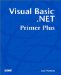# Review Questions

 1: What is an operand? A1: An operand is a subexpression that resolves to some value. For example, if you are adding two numbers, the addition operator requires two operands ”in this case, the two numbers to be added together. 2: What is meant by the term binary operator ? A2: If an operator is a binary operator, it requires two operands to perform its function. This is the general format for a binary operator: ` Operand1 Operator Operand2 ` For example, the addition operator is a binary operator and hence needs two operands. Therefore, to add the numbers 10 and 20, Operand1 becomes 10 , and Operand2 becomes 20 : ` 10 + 20 ` The result of this addition expression evaluates to 30. 3: Suppose you want to have a variable named Odd equal 1 if a number is odd and if the number is even. Assume that a variable named Number holds the value to test. Write the code that sets Odd correctly. A3: You need to write a single statement ` Odd = Number Mod 2 ` The Mod operator returns the remainder of division. Therefore, an odd number Mod 2 will yield 1 whenever Number is odd. 4: What is a shorthand operator? A4: Some operations are performed so often that Visual Basic .NET provides a shortened version of the operator. For example, incrementing a variable is normally done like this: ` number = number + 1 ` This is the shorthand operator for the same operation is: ` number += 1 ` Each of the arithmetic operators has a shorthand version. The base operator precedes the assignment operator: ` Operand1 Operator = Operand2 ` You simply replace Operator with the operator you want to use. For example, to subtract 5 from a variable named Result , you use this: ` Result -= 5 ` 5: What is operator precedence? A5: Operator precedence refers to the way in which complex expressions are resolved. For example, in the expression: ` Result = 5 * 10 + 50 /2 ` what does Result equal? If you didn't know anything about operator precedence, the answer would be ambiguous. However, because certain arithmetic operators are performed before others, you can determine that the answer should be 75 .Visual Basic .NET Primer Plus
ISBN: 0672324857
EAN: 2147483647
Year: 2003
Pages: 238
Authors: Jack Purdum

Similar book on Amazon